### Adding Two numbers using float and integer data

নিম্নের সোর্সকোডের মাধ্যমে পূর্ণসংখ্যাকে(Integer) এবং বাস্তব সংখ্যাকে(real number) কিভাবে যোগ করা যায় এবং তা প্রিন্ট(show) করা যায় তা শেখা যাবে । ভেরিয়েবল এবং ডাটা টাইপ সম্পর্কে বিস্তারিত বুঝার জন্য ভিজিট করুন:  http://www.cprogramming-bd.com/tutorial/c_tutorial_page_6.aspx

Problem: Write a program which performs addition on two numbers and display the result. Use float and Integer data type

Source Code:

#include<stdio.h>
main()
{
int number;
float amount;
//integer data representation
number=100+10;
//float data representation
amount =30.75 +  75.35;
printf("%d\n", number);
printf("%5.2f",amount);
}

Output:
110
106.10

<< Go to Index Page >>

1.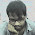//The problem solve by using "scanf"...the value will be calculate by manually.

#include
#include
main()
{
double a,b,number,amount;
number=100;
printf("Enter the value of 1st amount: \n");
scanf("%lf",&a);
printf("Enter the value of 2nd amount: \n");
scanf("%lf",&b);
amount=a+b;
printf("The number is: %lf\n",number);
printf("The amount is: %0.2lf\n",amount);
return 0;
}

2.3.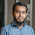#include"stdio.h"
main(){
float a,b,sum;
printf("Enter One number : \n");
scanf("%f",&a);
printf("Enter Another number : \n");
scanf("%f",&b);
sum=a+b;
printf("The sum of two numbers is %.2f \n",sum);
}

4.#include
main()
{
int a,b,sum=0;
scanf("%d",&a);
scanf("%d",&b);
sum=a+b;
printf("%d",sum);

}

5.#include
main()
{
int a,b,result;
printf("Enter the value of A\n");
scanf("%d",&a);
printf("Enter the value of B\n");
scanf("%d",&b);
result=a+b;
printf("The result is %d",result);

}

6.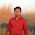7.8.# include
main ()
{
return 0;
}

9.# include
main ()
{
return 0;
}

10.#include"stdio.h"
main(){
float a,b,sum;
printf("Enter One number : \n");
scanf("%f",&a);
printf("Enter Another number : \n");
scanf("%f",&b);
sum=a+b;
printf("The sum of two numbers is %.2f \n",sum);
}

11.//adding two numbers in c++
#include
using namespace std;
main()
{
int a,b,c=0;
cout<<"Enter 1st element"<>a;
cout<<"Enter 2nd element"<>b;
c=a+b;
cout<<"The sum is "<<c;
}

12.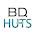#include

void main()
{
int firstN, secondN, totalN;
printf("Enter two numbers to add \n");
scanf("%d%d",&firstN,&secondN);
totalN = firstN + secondN;
printf("Total Number is= %d\n",totalN);

}

13.sonia ferdusi
id-201410285

#include
void main()
{
int a=13,b=13;
int sum=0;
cin("%d",&a);
cin("%d",&b);
sum=a+b;
cout("%d",&sum);
}

14.//adding two numbers in c++
#include
#include
#include

using namespace std;
main()
{
int a,b,sum=0;
cout<<"Enter 1st element"<>a;
cout<<"Enter 2nd element"<>b;
sum=a+b;
getch();
}
Bikash Chandra Sutrodhor
ID: 201410108
Batch: 45th

15.//adding two numbers in c++
#include
#include
#include
using namespace std;
main()
{
int a,b,c=0;
cout<<"Enter 1st element"<>a;
cout<<"Enter 2nd element"<>b;
c=a+b;
cout<<"The sum is "<<c;
getch();
return 0;
}
Sumonto Kumer Ghosh
ID : 201410848
Batch : 45th

16.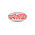//adding two numbers in c++

#include"stdio.h"
main()
{
int c,d,sum=0;
scanf("%d",&c);
scanf("%d",&d);
sum=c+d;
printf("%d",sum);

}

ID: 201411044
Batch: 45th

17.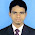main()
{
int a,b,sum:
cout<<"Enter Your First Value";
cin>>a;
cout<<"Enter Your Second Value";
cin>>b;
sum=a+b;
cout<<"Sum of Two Numbers is"<<sum;
cout<<sum<<endl;
}

18.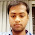Rubel(201410530)

#include
using namespace std;
int main()
{
int p, r, S;
cout<<"Enter two number to add\n";
cin >> p >> r;
S=p+r;
cout<<"Sum of entered numbers="<<S<< end1;
return 0;
}

19.fehir(201520314)

#include
using namespace std;
int main()
{
int a,b;
cin>>a>>b;
cout<<a+b;
return 0;
}

20.#include
using namespace std;

int main(){
int a, b;
cout<<"Enter two numbers:" ;
cin>>a>>b;

int result = a+b;

cout<<endl<<"The result is: " <<result <<endl;

return 0;
}

21.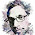Appel mahmud akib. Id:201510384

#include
using namespace std;
int main()
{
int a,b;
cin>>a>>b;
cout<<a+b<<endl;
}

22.#include
using namespace std;

int main ()
{
int a,b;
cout<<"Enter two number:" ;
cin>>a>>b;

int result =a+b;
cout <<"the result is: "<<result;
return 0;
}

23.#include
using namespace std;

int main ()
{
int a,b;
cout<<"Enter two number:" ;
cin>>a>>b;

int result =a+b;
cout <<"the result is: "<<result;
return 0;
}

24.#include
using namespace std;

int main ()
{
int a,b;
cout<<"Enter two number:" ;
cin>>a>>b;

int result =a+b;
cout <<"the result is: "<<result;
return 0;
}

25.#include
using namespace std;
int main()
{
int a,b;
cin>>a>>b;
cout<<a+b;
}

26.#include
using namespace std;
int main()
{
int a,b;
cin>>a>>b;
cout<<a+b;
}

27.#include
using namespace std;
int main()
{
int a,b;
cin>>a>>b;
cout<<a+b;
}

28.#include
using namespace std;
int main()
{
int num1, num2, result;
cout<<"Enter two numbers: ";
cin>>num1>>num2;
cout<<endl;
result=num1+num2;
cout<<"RESULT: "<<result<<endl;
}

29.#include
using namespace std;
int main()
{
int num1, num2, result;
cout<<"Enter two numbers: ";
cin>>num1>>num2;
cout<<endl;
result=num1+num2;
cout<<"RESULT: "<<result<<endl;
}

30.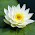using c++

#include

using namespace std;

double func(double i, double j)
{
return i+j;
}

int main()
{
double a, b;
cout << "Enter first value --:";
cin >> a;
cout << "Enter second value --:";
cin >> b;
cout << "Total value of two number --: " << func(a, b) << "\n";
return 0;
}

31.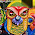Name: Tareq Aziz
ID : 201531141

#include

using namespace std;

int main() {
double a,b, total;
cout<<"Enter Two number"<>a>>b;
total=a+b;
cout<<"Total : "<< total;
}

32.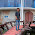#include

using namespace std;

int fun(int i, int j)
{
return i+j;
}
int main()
{
int i, j;
cout << "Enter first number: ";
cin >> i;
cout << "Enter second number: ";
cin >> j;
cout << "Addition between two number is: " << fun(i, j);
return 0;
}

33.34.using C++
#include

using namespace std;
int main()
{
int a,b;
int result;
cin>>a;
cin>>b;
result =a+b;
cout<<result;
return 0;
}

35.using C++
#include

using namespace std;
int main()
{
int a,b;
int result;
cin>>a>>b;
result =a+b;
cout<<result;
return 0;
}

36.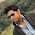using c++

#include
using namespace std;

int main()
{
int a,b,sum;
cout<<"Enter two number to add";
cin>>a>>b;
sum=a+b;
cout<<"sum of the number"<<sum;
return 0;
}

37.#include
using namespace std;

int main()
{
double A,B,SUM;

cout <<"Enter the number of A: "<< endl;
cin >>A;
cout <<"Enter the number of B: "<< endl;
cin >>B;
SUM = A+B;
cout <<"The sum is: "<<SUM<< endl;
return 0;
}

id:201510660

38.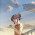#include
using namespace std;

int main()
{
double A,B,SUM;

cout <<"Enter the number of A: "<< endl;
cin >>A;
cout <<"Enter the number of B: "<< endl;
cin >>B;
SUM = A+B;
cout <<"The sum is: "<<SUM<< endl;
return 0;
}

id: 201510992

39.#include
using namespace std;

int main()
{
double A,B,SUM;

cout <<"Enter the number of A: "<< endl;
cin >>A;
cout <<"Enter the number of B: "<< endl;
cin >>B;
SUM = A+B;
cout <<"The sum is: "<<SUM<< endl;
return 0;
}

40.#include
#include
Void main()
{
int a,b,c;
cout<<"Enter two numbers"<>a>>b;
c=a+b;
cout<<"Sum of two numbers are"<<c;
cout<<c<<endl;
getch();
}

41.#include
#include
Void main()
{
int a,b,c;
cout<<"Enter two numbers"<>a>>b;
c=a+b;
cout<<"Sum of two numbers are"<<c;
cout<<c<<endl;
getch();
}

42.#include
using namespace std;
int main()
{
int a,b,sum;
cout<<"Enter Integer values"<>a>>b;
sum=a+b;
cout<<"Sum of integer values is :"<>c>>d;
cout<<"Sum of Float values is :"<<add<<endl;
}

43.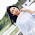#include
using namespace std;
int main(){
int a,b,sum=0;
cout<<"enter the first number="<>a;
cout<<"enter the second number="<>b;
sum+=a+b;
cout<<"the sum of the two number is ="<<sum<<endl;
}

44.#include
int main(){
int a;
float b,sum;
printf("enter the integer number=");
scanf("%d",&a);
printf("enter the float number=");
scanf("%f",&b);
sum=a+b;
printf("%.2f\n",sum);

}

45.mausumi sumi

#include
using namespace std;
int main(){
int a,b,c;
cout<<"enter two number to add\n";
cin>>a>>b;
c=a+b;
cout<<"sum of entered number="<<c<<endl;
}

46.#include
int main()
{
int a,b,sum;
scanf("%d %d",&a,&b);
sum=a+b;
printf("%d",sum);
return 0;

}

47.#include
int main()
{
int a,b,sum;
scanf("%d %d",&a,&b);
sum=a+b;
printf("%d",sum);
return 0;

}

48.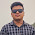#include
int main()
{
int Number1, Number2, sumOfTwoNumbers;

printf("Enter two integers: ");

scanf("%d %d", &Number1, &Number2);

sumOfTwoNumbers = Number1 + Number2;

printf("%d + %d = %d", Number1, Number2, sumOfTwoNumbers);

return 0;
}

49.#include
#include

void main( )
{
float n1, n2, sum;
printf("Enter first number: ");

scanf("%f", &n1);

printf("Enter second number: ");

scanf("%f", &n2);

sum=n1+n2;

printf("Sum of two numbers= %f", sum);

}

50.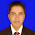ID: 201820244
Batch:58th
Section: B

#include
int main(){
float x,y,result;
printf("Enter One number : \n");
scanf("%f",&x);
printf("Enter Another number : \n");
scanf("%f",&y);
result=x+y;
printf("The sum of two numbers is %.2f \n",result);
}

51.ID-201820298
58 BATCH

https://we.tl/t-V7Te3itBME

52.ID#201830546

#include
using namespace std;
main()
{
int number,a,b;
float amount,x,y;
cout<< "Enter two integer number";
cin>> a>> b>>endl;
amount=a+b;
cout<< "Sum of two integer no is :"<> x>> y>>endl
amount=x+y;
cout<< "Sum of two float no is :"<<amount;

}

53.Name : Md. Zoynul Abedin
ID : 201820591
Batch : 58th

** Adding Two numbers using float and integer data using C language.

#include
int main()
{
int Number1, Number2, sumOfTwoNumbers;

printf("Enter two integers: ");

scanf("%d %d", &Number1, &Number2);

sumOfTwoNumbers = Number1 + Number2;

printf("%d + %d = %d", Number1, Number2, sumOfTwoNumbers);

return 0;
}

54.ID: 201330475
Ibrahim Khalil

#include
using namespace std;

int main(){
int a;
float b, result;
cout<<"Enter first numbers:" ;
cin>>a;
cout<<"Enter second numbers:" ;
cin>>b;

result = a+b;

cout<<endl<<"The result is: " <<result <<endl;

return 0;
}

55.ID:201630992
Sijar khan

#include
using namespace std;

{

public:
int a,b;
float x,y,sum=0.00;

{
cout<<"Enter how many number: ";
cin>>a>>b;

cout<<"Enter how many number: ";
cin>>x>>y;

}
{

}
int display()
{

return sum = a + b;

}
float display1()
{
return sum = x + y;
}

};

int main()
{